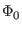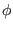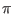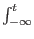Next: Josephson Junction (Tunnel Junction Up: Josephson Junctions Previous: Josephson Junctions   Contents   Index

### Josephson Junction Description

The Josephson junction device has unique behavior which complicates simulation with a SPICE-type simulator. Central is the idea of phase, which is a quantum-mechanical concept and is generally invisible in the non-quantum world. However with superconductivity, and with Josephson junctions in particular, phase becomes not only observable, but a critical parameter describing these devices and the circuits that contain them.

Without going into the detailed physics, one can accept that phase is an angle which applies to any superconductor. The angle is a fixed value anywhere on the superconductor, unless current is flowing. Flowing current produces magnetic flux, and magnetic flux produces a change in phase. One can express this as follows:

LI = flux =(/2)

Here, L is the inductance,is the magnetic flux quantum (Planck's constant divided by twice the electron charge) andis the phase difference across the inductor. The supercurrent flowing in a Josephson junction is given by

I = Icsin()

where Ic is the junction critical current, andis the phase difference across the junction. The junction phase is proportional to the time integral of junction voltage:= (2/)V(t)dt

The important consequence is that the sum of the phase differences around any loop consisting of Josephson junctions and inductors must be a multiple of 2. This is due to the requirement that the superconducting wave function be continuous around the loop. Further, if the loop phase is not zero, it implies that a persistent current is flowing around the loop, and that the magnetic flux through the loop is a multiple of the flux quantum.

We therefor observe that in a circuit containing loops of Josephson junctions and inductors, which includes about all useful circuits:

1. The DC voltage across each Junction or inductor is zero.
2. The DC current applied to the circuit splits in such a manner as to satisfy the phase relations above.

Without any built-in concept of phase, it would appear to be impossible to find the DC operating point of a circuit containing Josephson junctions and inductors with a SPICE simulator. However, there are ways to accomplish this.

The time-honored approach, used successfully for many years, is to skip DC analysis entirely. One generally is interested only in transient analysis, describing the time evolution of the circuit under stimulus, and a DC analysis would only be necessary to find the initial values of circuit voltages and currents. Instead of a DC analysis, what is done is every voltage and current source starts at zero voltage or current, and ramps to the final value in a few picoseconds. The transient analysis is performed using the use initial conditions'' (uic'') option, where there is no DC operating point analysis, and transient analysis starts immediately with any supplied initial condicions (which are not generally given in this case). By ramping up from zero, the phase condition around junction/inductor loops is satisfied via Kirchhoff's voltage law. Actually, this ensures that the loop phase is constant, but it is zero as we started from zero. Initially, there is no trapped flux'' in the inductor/junction loops, so assumption of zero phase is correct. Thus the prescription is to ramp up all sources from zero, use the uic option of transient analysis, and wait for any transients caused by the ramping sources to die away before starting the real'' simulation. The ramping-up effectively replaces the DC operating point analysis.

The second approach is to use phase-mode DC analysis (see 2.7.3.1), which is used in WRspice transparently when Josephson junctions are present. This applies to explicit DC analysis as well as operating point analysis. Further, this enables AC and similar small-signal analysis with Josephson junctions in WRspice.